# Test Prep Plan - Take a practice test

Take this practice test to check your existing knowledge of the course material. We'll review your answers and create a Test Prep Plan for you based on your results.
How Test Prep Plans work
1
2Based on your results, we'll create a customized Test Prep Plan just for you!
3Study smarter
Study more effectively: skip concepts you already know and focus on what you still need to learn.

# FTCE Math: Graphing Derivatives Chapter Exam

Exam Instructions:

Choose your answers to the questions and click 'Next' to see the next set of questions. You can skip questions if you would like and come back to them later with the yellow "Go To First Skipped Question" button. When you have completed the practice exam, a green submit button will appear. Click it to see your results. Good luck!

### Page 1

#### Question 1 1. What is the slope of a line normal to f(x)?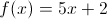#### Question 2 2. The derivative of the following graph is _____ at point A.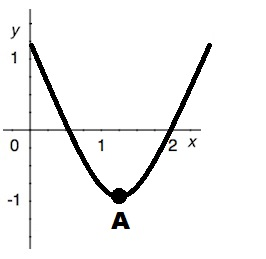#### Question 3 3. The graph shown is an example of a function that: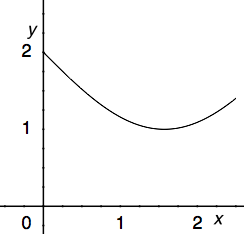#### Question 4 4. The graph shown is an example of a function that: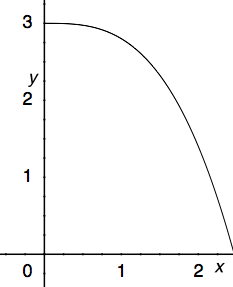#### Question 5 5. Which of the following is a local minimum?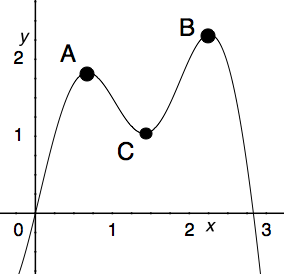### Page 2

#### Question 6 6. Which could be the graph of the derivative of the function below?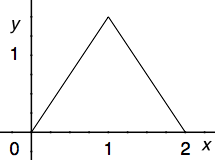#### Question 7 7. Consider the function f(x) below. At x = 1 is this function concave up, concave down, or an inflection point?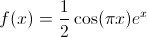#### Question 9 9. The graph shown is an example of a function that: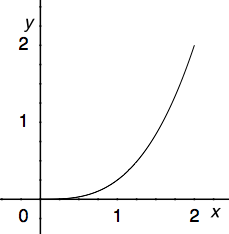#### Question 10 10. Which of the following BEST describes point B?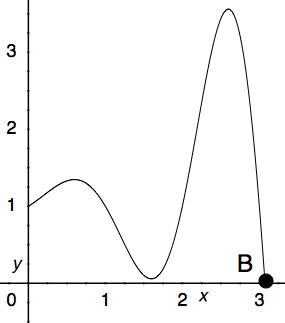### Page 3

#### Question 11 11. Over the region shown, point A is known as _____.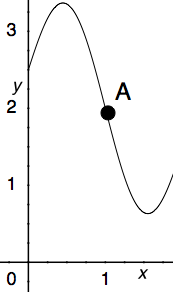#### Question 12 12. Where is the global maximum for the function y=f(x) below between x=0 and x=2?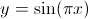#### Question 13 13. What is the equation of the line normal to f(x) at the point x=1?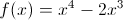#### Question 14 14. Which of the following BEST describes point A?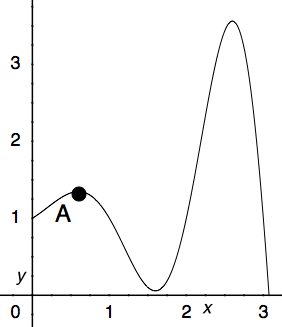### Page 4

#### Question 16 16. The derivative of the following graph is _____ at point D.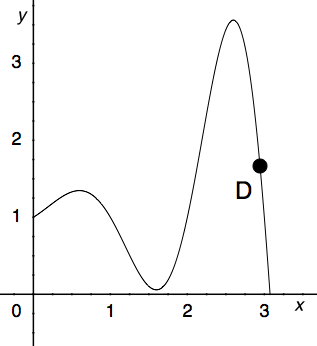#### Question 17 17. What is the equation of the line tangent to f(x) at the point x=0?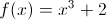#### Question 18 18. Which of the following BEST describes point C?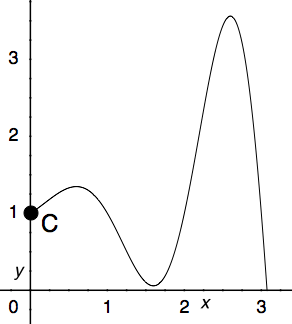#### Question 20 20. What is the slope of the line normal to f(x) at x=1?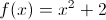### Page 5

#### Question 22 22. What is the global minimum for the function y=f(x) below between x=0 and x=2?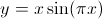#### Question 23 23. Between x=1 and x=3, the following function _____.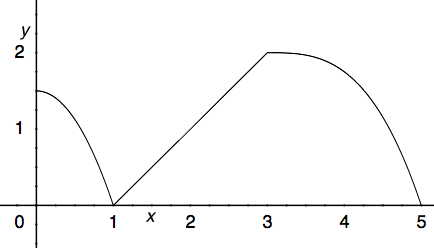#### Question 25 25. Consider the function f(x) below. At x = 2 is this function concave up, concave down, or an inflection point?### Page 6

#### Question 26 26. What is value of y at the finite local maximum for the function y=f(x) below?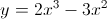#### Question 27 27. Consider the function g(x) below. At x = 0 is this function concave up, concave down, or an inflection point?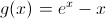#### Question 29 29. What is the global minimum for the function y=f(x) below between x=0 and x=2?#### Question 30 30. What is the slope of the tangent to r(s) at s = 0?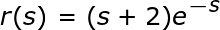#### FTCE Math: Graphing Derivatives Chapter Exam Instructions

Choose your answers to the questions and click 'Next' to see the next set of questions. You can skip questions if you would like and come back to them later with the yellow "Go To First Skipped Question" button. When you have completed the practice exam, a green submit button will appear. Click it to see your results. Good luck!

Support